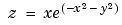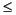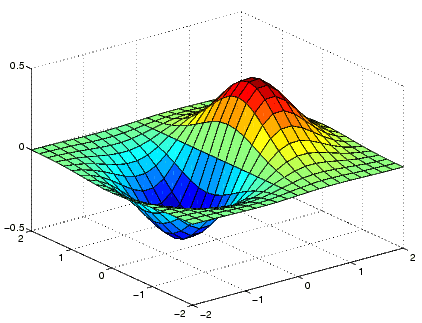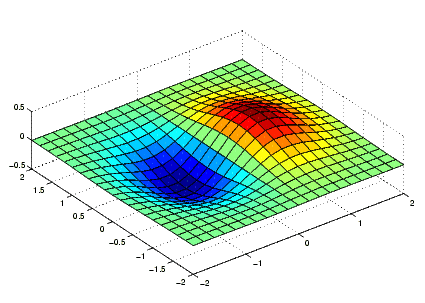MATLAB Function Referencedaspect

Set or query the axes data aspect ratio

Syntax

• ```daspect
daspect([aspect_ratio])
daspect('mode')
daspect('auto')
daspect('manual')
daspect(axes_handle,...)
```

Description

The data aspect ratio determines the relative scaling of the data units along the x-, y-, and z-axes.

```daspect ``` with no arguments returns the data aspect ratio of the current axes.

```daspect([aspect_ratio]) ``` sets the data aspect ratio in the current axes to the specified value. Specify the aspect ratio as three relative values representing the ratio of the x-, y-, and z-axis scaling (e.g., `[1 1 3]` means one unit in `x` is equal in length to one unit in `y` and three units in `z`).

```daspect('mode') ``` returns the current value of the data aspect ratio mode, which can be either `auto` (the default) or `manual`. See Remarks.

```daspect('auto') ``` sets the data aspect ratio mode to `auto`.

```daspect('manual') ``` sets the data aspect ratio mode to `manual`.

```daspect(axes_handle,...) ``` performs the set or query on the axes identified by the first argument, `axes_handle`. When you do not specify an axes handle, `daspect` operates on the current axes.

Remarks

`daspect` sets or queries values of the axes object `DataAspectRatio` and `DataAspectRatioMode` properties.

When the data aspect ratio mode is `auto`, MATLAB adjusts the data aspect ratio so that each axis spans the space available in the figure window. If you are displaying a representation of a real-life object, you should set the data aspect ratio to `[1 1 1]` to produce the correct proportions.

Setting a value for data aspect ratio or setting the data aspect ratio mode to `manual` disables the MATLAB stretch-to-fill feature (stretching of the axes to fit the window). This means setting the data aspect ratio to a value, including its current value,

• ```daspect(daspect)
```

can cause a change in the way the graphs look. See the Remarks section of the `axes` description for more information.

Examples

The following surface plot of the functionis useful to illustrate the data aspect ratio. First plot the function over the range -2x2, -2y2,

• ```[x,y] = meshgrid([-2:.2:2]);
z = x.*exp(-x.^2 - y.^2);
surf(x,y,z)```

Querying the data aspect ratio shows how MATLAB has drawn the surface.

• ```daspect
ans =
4  4  1
```

Setting the data aspect ratio to `[1 1 1]` produces a surface plot with equal scaling along each axis.

• ```daspect([1 1 1])```

See Also

`axis`, `pbaspect`, `xlim`, `ylim`, `zlim`

Setting the Aspect Ratio and Axis Limits for related functions

Axes Aspect Ratio for more informationcylinder datacursormode© 1994-2005 The MathWorks, Inc.